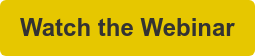# Compute Grid Spacing for a Given Y+

## Improve CFD Accuracy with Correct Mesh Resolution

It's important that your mesh near the wall is properly sized to ensure accurate simulation of the flowfield. This calculator computes the height of the first mesh cell off the wall required to achieve a desired Y+ using flat-plate boundary layer theory.

## Input

freestream velocity (m/s)
freestream density (kg/m3)
dynamic viscosity (kg/m s)
reference length (m)
desired y+

## Output

wall spacing (m)
Reynolds number
Note: -1 indicates an input error

### How Do You Define a Good Grid

Computational fluid dynamics (CFD) is essentially a series of approximations; the role of the practitioner is managing the magnitude of the errors in these approximations. In CFD, one of the most basic approximations lies in the type of cells used for the simulation. This article will address some of the potential pitfalls encountered with a generic cell-centered finite volume scheme.### How to Improve Accuracy Using Pointwise’s Mesh Adaptation

This webinar, “Towards Mesh Adaptation,” introduces a feature currently in development for mesh adaptation. It accepts from the flow solver a point cloud on which target cell sizes are specified. This point cloud is used as a clustering source in Pointwise to adapt both the volume and surface meshes.## Calculate Grid Spacing on iPhone and Android™

Now you can compute wall spacing while at your desk or while on the road with the Y+ Calculator for iPhone, iPad, or Android. This free app is a handy tool for calculating the grid spacing at a wall to achieve a target y+ value for viscous computational fluid dynamics (CFD) computations. Simply specify the flow conditions, the desired y+ value, and compute your grid spacing. The Y+ Calculator converts between SI and Imperial units.

*Image courtesy of Tecplot

## The Math

These computations are based on flat-plate boundary layer theory from Frank M. White's Fluid Mechanics 5th edition, page 467.

${\mathrm{Re}}_{x}=\frac{\rho {U}_{\infty }L}{\mu }$

${C}_{f}=\frac{0.026}{{{\mathrm{Re}}_{x}}^{1/7}}$

${\tau }_{wall}=\frac{{C}_{f}\rho {{U}_{\infty }}^{2}}{2}$

${U}_{fric}=\sqrt{\frac{{\tau }_{wall}}{\rho }}$

$\Delta s=\frac{{y}^{+}\mu }{{U}_{fric}\rho }$